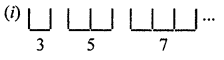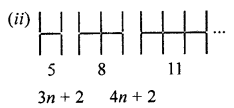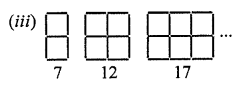## ML Aggarwal Class 7 Solutions for ICSE Maths Chapter 8 Algebraic Expressions Ex 8.4

Question 1.
Observe the patterns of shapes of letters formed from line segments of equal lengths:If n shapes of letters are formed, then write the algebraic expression for the number of line segments required for making these n shapes in each case.
Solution:2 × 1 + 1 = 2 + 1 = 3
2 × 2 + 1 = 4 + 1 = 5
2 × 3 + 1 = 6 + 1 = 7
2 × n + 1 = 2n + 1 ; n = 1,2, 3,5 = 3 × 1 + 2 = 3 + 2 = 3 × 1 + 2
8 = 3 × 2 + 2 = 6 + 2 = 3 × 2 + 2
11 = 3 × 3 + 2 = 9 + 2 = 3 × 3 + 2
Generalising, we get 3 × n + 2
Hence, 3n + 2

Question 2.
Observe the pattern of shapes of digits formed from line segment of equal lenghts:If n shapes of digits are formed, then write the algebraic expression for the number of line segments required for making these n shapes in each case.
Solution:4 = 3 × 1 + 1 = 3 + 1
7 = 3 × 2 + 1 = 6 + 1
10 = 3 × 3 + 1 = 9 + 1
Generalising, we get 3n+ 16 = 5 × 1 + 1
11 = 5 × 2 + 1
16 = 5 × 3 + 1
Generalising, we get 5n + 15 × 1 + 2 = 7
5 × 2 + 2 = 12
5 × 3 + 2 = 17
Generalising, we get 5n + 2

Question 3.
If a pattern consists of n shapes formed by line segments of equal lengths and the number of line segments required to make n shapes is given by the algebraic expression:
(i) 2n + 1
(ii) 3n + 1
(iii) 3n + 2
(iv) 5n + 1
(v) 5n + 2
(vi) 4n + 3
then find the number of line segments required to form 5, 10 and 100 shapes in each case.
Solution:
(i) 2n + 1
Taking n = 5, 10, 100, we get
2 × 5 + 1 = 11
2 × 10 + 1 = 21
2 × 100 + 1 = 201
(ii) 3n + 1
Taking n = 5, 10, 100, then we get
3 × 5 + 1 = 16
3 × 10 + 1 = 31
3 × 100 + 1 = 301
(iii) 3n + 2
Taking n = 5, 10, 100, then we get
3 × 5 + 2 = 17
3 × 10 + 2 = 32
3 × 100 + 2 = 302
(iv) 5n + 1
Taking n = 5, 10, 100, then we get
5 × 5 + 1 = 26
5 × 10 + 1 = 51
5 × 100 + 1 = 501
(v) 5n + 2
Taking n = 5, 10, 100, then we get
5 × 5 + 2 = 27
5 × 10 + 2 = 52
5 × 100 + 2 = 502
(vi) 4n + 3
Taking n = 5, 10, 100, then we get
4 × 5 + 3 = 23
5 × 10 + 3 = 53
5 × 100 + 3 = 503